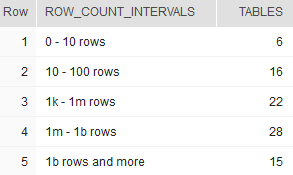## Number of tables by the number of rows in Snowflake

Article for:

If you want to get an overview on how many rows tables in your database hold one way is to count them by row intervals. This query returns number of tables by the number of their rows grouped into predefined intervals.

## Query

``````select
distinct
row_count_intervals,
count ("table_name") over (partition by row_count_intervals) as tables
from
(
select t.table_schema || '.' ||  t.table_name as "table_name",
case when t.row_count > 1000000000 then '1b rows and more'
when t.row_count > 1000000 then '1m - 1b rows'
when t.row_count > 1000 then '1k - 1m rows'
when t.row_count > 100 then '100 - 1k rows'
when t.row_count > 10 then '10 - 100 rows'
else  '0 - 10 rows' end as row_count_intervals,
t.row_count
from information_schema.tables t
where t.table_type = 'BASE TABLE'
order by t.row_count
) y;
``````

## Columns

• row_count_intervals - predefined row count intervals:
• 0 - 10 rows
• 10 - 100 rows
• 100 - 1k rows
• 1k - 1m rows
• 1m - 1b rows
• 1b rows and more
• tables - number of tables that row count falls in that interval

## Rows

• One row represents one interval
• Scope of rows: all row count intervals that appear in the database
• Ordered by from smallest tables to the largest

## Sample results

Here is a number of tables by row count in SNOWFLAKE_SAMPLE_DATA database aggregated into predefined intervals.